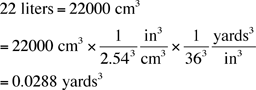Name: Kathy Who is asking: Parent Level of the question: All Question: I am trying to find out how many quarts of dirt there are in a yard of dirt. The local flower store sells bags of dirt that are 20 quarts or 22 liters for \$1.25 and another store sells a yard of dirt for \$25.00. Which is the better deal? Kathy Hi Kathy, I am going to use 22 liters as the volume of the bag rather than 20 quarts for two reasons. First the American and British quarts are different sizes and I don't know which to use and secondly the conversion between length measurements and volume measurements is simpler in the metric system than it is in the American or Imperial systems. The one conversion factor I remember between the two systems is that there are 2.54 centimeters in an inch. The conversion between length and volume in the metric system is that one liter is 1000 cubic centimeters (cm3). Thus 22 liters is 221000 = 22000 cm3 and henceThus at \$25.00 per cubic yard 22 liters of dirt would cost \$25.000.0288 = \$0.72 Hence purchasing the soil by the yard is a better deal. Penny